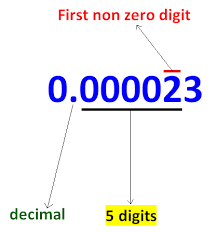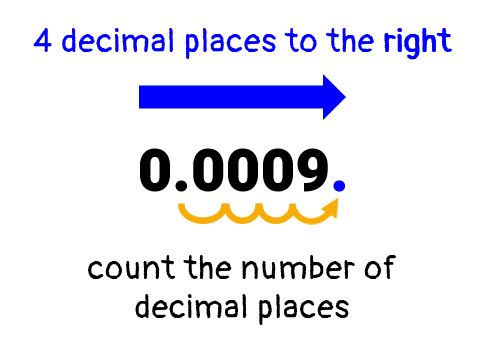# Standard Form Calculator

Enter the number in the input box to write in standard form/Scientific E-Notation/Engineering Notation using our standard form calculator (converter)/standard notation.## Standard Form Calculator

The standard form calculator is used to convert the numbers into standard form by placing the decimal value in the number. It converts a long number into an easily readable standard form.

It is a write in standard form calculator which takes the number from the user and convert to standard form.

In this content, we will explain what standard form is, how to use our standard form calculator, and how to calculate standard form as well.

## How to use standard form calculator?

To use this standard notation calculator, follow the below steps:

• Enter the number in the given input box.
• Press the Calculate button to see the result.
• You can reset the values by using the Reset

This standard form equation calculator will instantly show you the converted standard form of the given number. You can also use our scientific notation calculator and scientific notation converter to calculate the scientific notations.

## What is standard form?

If you are wondering what is standard form in math, you are at right place.

Standard form is used to reduce the difficulty in reading very large or very small numbers. Standard form of a number is any number between 1.0 and 10.0 multiplied by power 10, For example, 1.2× 102

For a demonstration of standard form, take a look at below examples:

Number: 85500000000000

Standard form: 8.55 × 1013

Number: 0.000458912

Standard form: 4.58 × 10-4

## How to write in standard form?

You can use our convert to standard form calculator to calculate the standard form of any number. However, we will explain how you can convert a number to standard form manually. To convert a number to standard form, follow the below steps:

1. Write down the number.
2. Identify the decimal point in the number. If there is no decimal point in the given number, it is considered as at the right side of the number after the last digit.
3. After identifying the decimal point, move the decimal to the first non-zero digit in the number.4. Count the total number of digits you have moved the decimal point. Multiply the number with 10 and raise the power of 10 with the total number of digits decimal have moved. If the decimal is moved from right to left, power will be positive, and if the decimal is moved from left to right, power will be negative.

Example:

Convert 0.0009 to the standard form.

Solution:

Follow the steps to find the standard form of the given number.

Step 1: Write down the number.

0.0009

Step 2: Identify the decimal point in the number. You can see the decimal point is lying after 4 digits from the left side.

Step 3: After identifying the decimal point, move the decimal to the first non-zero digit in the number.

It will become 9. Because there is no non-zero digit after 9, we don’t need to write the decimal point after 9.Step 4: Count the total number of digits you have moved the decimal point. We have moved the decimal 4 places further. Multiply the number with 10 and raise the power of 10 with the total number of digits decimal have moved. As we have moved the decimal point from left to right, the power will be negative.

9 × 10-4

So, the standard form of the number 0.0009 is 9 × 10-4.

Examples:

 25*10^6 in standard form 2.5 x 10^7 5004300 in standard form 5.0043 x 10^6 0.00147 in standard form 1.47 x 10^-3 0.884 in standard form 8.84 x 10^-1 234.543 x 10^2 in standard form 2.34543 x 10^4
1. Mathsisfun.com. Standard Form.
2.  Splashlearn.com. What Is Standard Form? - Definition, Facts & Example.
3. Math Only Math, Standard Form Of A Number | Numeral In Standard Form.
4.  Amathsdictionaryforkids.com. Standard Form ~ Reference By Jenny Eather.
5. Revisionmaths.com.Standard Form - Mathematics GCSE Revision.
6. Varsitytutors.com. Standard Form Of A Line.